A New Non-Doppler Redshift
Paul Marmet, Herzberg Institute of Astrophysics
National Research Council, Ottawa, Ontario, Canada, K1A 0R6
( Last checked 2018/01/16 - The estate of Paul Marmet )
Updated from: Physics Essays, Vol. 1, No: 1, p. 24-32, 1988
<><><><><><><><><><><><>
Return to:  List of Papers on Web Sites

Eine deutsche Übersetzung dieses Artikels finden Sie hier.

Abstract
It is known that many astronomical observations cannot be explained by means of the ordinary Doppler shift interpretation.  The mere examination of a recent catalog of objects having very large redshifts shows that among 109 quasi-stellar objects for which both absorption and emission lines could be measured, the value of the absorption redshift of a given object is always different from the one measured in emission for the same object.  It is clear that such results cannot be explained as being due solely to a Doppler redshift.
A new mechanism must be looked for, in order to explain those inconsistent redshifts and many other observations related to the “redshift controversy”.
It is possible to calculate a very slight inelastic scattering phenomenon compatible with observed redshifts using electromagnetic theory and quantum mechanics, without the need to introduce ad hoc physical hypotheses.
A careful study of the mechanism for the scattering of electromagnetic radiation by gaseous atoms and molecules shows that an electron is always momentarily accelerated as a consequence of the momentum transfer imparted by a photon.  Such an acceleration of an electric charge produces bremsstrahlung.
It is shown in the present work that this phenomenon has a very large cross section in the forward direction and that the energy lost by bremsstrahlung causes a slight redshift.  It is also demonstrated that the relative energy loss of the electromagnetic wave for blackbody radiation, such as for many celestial objects, follows the same Dn/n =  constant law as if it were a Doppler law.
This redshift appears indistinguishable from the Doppler shift except when resonant states are present in the scattering gas.  It is also shown that the lost energy should be detectable mostly as low frequency radio waves.  The proposed mechanism leads to results consistent with many redshifts reported in astrophysical data.

1. Introduction
Astrophysical observations show that the electromagnetic radiation originating from cosmological objects is often redshifted.  Except for some hypothesis such as assuming that it is a gravitational redshift, this has always been interpreted as a Doppler shift.  To date, the interaction of light with interstellar gas has not been seriously considered as a possible mechanism responsible for the observed redshift because no known forward scattering process could be demonstrated to lead to an effect compatible with common astronomical observations.  The redshift observed in astronomy that agrees with a shift of Doppler origin, follows the relationship:(1)
where Dn is the change in frequency of the radiation and n is the frequency of the emitted light.
Thomson scattering however does not lead to Eq. (1).  In this case, electrons accelerated by the transverse electric field of the incident electromagnetic radiation emit radiation due to their transversal acceleration.  For example, the polarized blue radiation scattered by the daytime sky results from the transverse acceleration of bound electrons by visible light.  It is well known that the cross section leading to such scattering increases very rapidly as a function of frequency n and therefore cannot lead to a red shift following Eq. (1).
Let us now consider the photon momentum in the direction of the propagation of the wave. It is this momentum which produces the Compton effect.  In this case, the momentum transfer from the photon to the electron is taken into account.  However, no one has ever fully taken into account the bremsstrahlung resulting from the momentum transferred to an electric charge, when the energy of the electromagnetic radiation is imparted to electrons or atoms. Although Boekelheide (1) and Cavanaugh (2) observed energy losses at very high energy due to relativistic effects on free electrons called "double Compton scattering", no one (3) has found a full solution to the S-matrix that could describe electromagnetic wave interaction on atom at very low energy.  It is this low energy interaction which is interesting here.
Maxwell’s equations predict that radiation is emitted as a consequence of the change of velocity (acceleration) of the electron impinged on, due to momentum transfer.  That point has been taken into account in quantum electrodynamics as explained by Jauch and Rohrlich (3) who show that such a phenomenon always exists, as seen in their statement:
"This bremsstrahlung or deceleration radiation with the emission of a single photon is a well defined process only within certain limits: The simultaneous emission of very soft photons – too soft to be observed within the accuracy of the energy determination of the incident outgoing electron can never be excluded. In fact, this radiation is always present even in the so-called elastic scattering (3) ."
In this paper, we consider this problem at very low energy (visible light and lower energy) where classical considerations are still mostly valid.  We further consider the case of photon scattering on atoms at an extremely low atom density, which is a condition prevailing in outer space. In the usual treatment of the Compton effect, bremsstrahlung is neglected.  In these circumstances, it is known that the change Dl in wavelength is given by: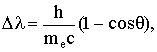(2)
where h = Planck’s constant, me = mass of the electron, c = velocity of light in vacuum and q = scattering angle.
From Eq. (2), we must notice that at any angle of scattering, bremsstrahlung is completely neglected.  However, the electron is accelerated during the scattering.  In order to illustrate the basic principle leading to an energy loss due to bremsstrahlung, let us examine the case of 90o Compton scattering on a free electron which is initially at rest.  The photon momentum transferred to the electron is such that the collision imparts motion to it.  Since the electron, initially at rest, becomes in motion after the impact, somehow it must have been accelerated.
According to electromagnetic theory, any accelerated charge must emit bremsstrahlung.  Since the Compton electron has been accelerated, it must emit bremsstrahlung.  Although the energy emitted due to such acceleration is extremely small, it is not zero and should not be neglected as done at low energy.  It will be seen that this energy loss adds a slight correction to Eq. (2).  The case of interaction at q = 0 requires special considerations.  It can be considered either as an extreme case of Compton scattering (q = 0) or better as the simple transmission of radiation through the particles of a gas.  In the latter case, the scattering angle is essentially zero degrees, but the physical reality of interaction with atoms is evident because the observed average speed of light is reduced in gases.
This reduced speed of light in gases is frequently calculated with the help of the index of refraction.  In this paper, that parameter will be calculated as the group velocity and will be considered in more detail below.  The interaction during transmission (or the scattering angle q = 0) is the only one that will be treated in this paper, since it leads to measurable predictions of light traveling through space.
In order to be able to evaluate the energy loss due to such a phenomenon, one needs to calculate different parameters such as the time of coherence of the electromagnetic radiation, the index of refraction of gases, and several other quantities.  These parameters will be calculated in Appendices A and B.

2. Bremsstrahlung Due to Axial Momentum Transfer.
2.1 Basic Mechanism
In the corpuscular description of light, it is considered that a particle, called a photon, is emitted from an excited state after its average lifetime Dt.  This corresponds in electromagnetic theory to an oscillating dipole continuously emitting coherent electromagnetic radiation whose amplitude is uniformly damped to a fraction 1/e of its initial value during the lifetime of the excited state.  This lifetime is equivalent to the time of coherence of the radiation.  During absorption, the phenomenon is reversed and the time of coherence Dt becomes the time of interaction of the wave with the atom.
This time of interaction Dt characterizes the wave as its time of coherence.  In the case of the emitter, this characteristic can be considered as the average time taken by the emitter to emit a photon.  When using a wave description, it is physically possible to deduce the time of coherence which is the pulse duration of the wave packet.  Therefore Dt appears to belong not only to the emitter but also to the wave.
One must recognize another property of the waves: Electromagnetic waves not only have energy but also momentum.  If one considers that the electromagnetic wave imparts its energy during an effective pulse duration Dt, one must remember that it also imparts its momentum during that time.
When an atom absorbs a wave train (photon), the total momentum is conserved and therefore must appear within the atom.  The electron is therefore accelerated due to the momentum transfer from the photon to the mobile electron.  The much smaller fraction of momentum imparted to the nucleus is much smaller and can be neglected here.  The electron is then accelerated during a length of time equal to the time of coherence of the absorbed radiation.  After absorption, the energy stays in the atom for a short interval of time which explains the apparent reduced speed of light in gases.  Finally, the mechanism is reversed and the energy absorbed of the wave is re-emitted.  This short time interval accounts for the index of refraction of gases as explained in Appendix B.  This selective forward reemission is obviously necessary to explain the transparency of gases and the straight-line propagation of light through them.

2.2 Transmission with Bremsstrahlung.
It is known that, according to electrodynamics, any accelerated electron must emit radiation.  Let us recall that we consider here an electron that is accelerated due to the axial momentum transfer of electromagnetic radiation.  Classical electromagnetic theory used here to treat the acceleration of an electron should lead to an excellent approximation.  This is because the same classical energy leads to an equally excellent answer when we calculate, at a similar energy, the electron inside an atom related to the Thompson scattering or Compton effect.
We must realize that classical considerations are valid here because of the "Correspondence Principle" (4).  The Correspondence Principle can be applied here because one deals with very low energy electromagnetic radiation polarizing a continuum of atomic hydrogen.  A better proof of validity of these semi classical considerations will be given in Appendix B using an experimental value with the value deduced from this theory (at similar energies).
Let us calculate the energy radiated due to the photon momentum transfer given to the electron.  The total power radiated by an accelerated charge e is: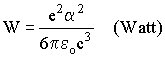(3)
where the acceleration a=Dv/Dt .
The time of coherence Dt is calculated in Appendix A.  The power radiated is: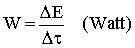(4)
Equations (3) and (4) yield(5)
where DE = energy radiated (Joule), e = electron charge (Coulomb), Dv change of velocity of electron due to momentum transfer (m/s), Dt = time during which the electron is accelerated (s), eo = permittivity of vacuum (F/m) and c = velocity of light in vacuum (m/s).
The momentum P of the wave train is:(6)

where n is the frequency of the incoming radiation.  Since this phenomenon is transferred to the electron, the change in velocity (Dv=P/m) gives: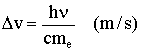(7)
where me is the electron mass.  Substituting Eq. (7) in Eq. (5), one finds that the energy radiated due to the absorption and the reemission of a photon by an electron is:(8)
In Appendix A, the Equation (A18) describes the time of coherence Dt (pulse duration) of the wave packet issued from blackbody radiation. We find: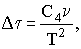(9)
where C4= 3.71 ´ 10-23 (s2K2).
Combining Eqs. (8) and (9) gives: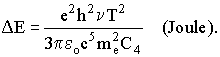(10)
Equation (10) gives the amount of bremsstrahlung radiated due to momentum transfer of radiation colliding with electrons.  Let us calculate the relative energy loss R in such a case.  From Eq. (10) and the energy of the wave train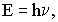(11)
we obtain: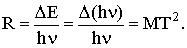(12)
where M=e2h/(3peoc5me2C4).
Numerically, we calculate that M=2.73 ´ 10-21 (K-2).
This energy loss can be applied to either bound or free electrons, but the cross sections differ greatly between these two cases, as shown in Appendix B.

2.3 Characteristics of the Energy Loss Equation.
It is interesting to note that in Equation (12), the relative energy loss is independent of the frequency n of the incoming radiation in the case stated (blackbody radiation).  Therefore, the whole spectrum will undergo a constant relative displacement in energy toward lower frequencies.  This displacement of the spectrum is exactly similar to the redshift produced when a source of radiation recedes from the observer (Doppler effect).  For example, the fractional redshift (5) for astronomical objects can be described by the fractional redshift constant: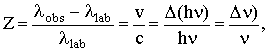(13)
where n is the radial component of the receding velocity of the light source.
Since the relative energy loss is independent of n in both cases, the new redshift described by Eq. (12) is undistinguishable from the Doppler redshift described by Eq. (13) in the energy range studied.  As a consequence, all absorption lines of a blackbody spectrum will be redshifted with the same dependence as Z as long as they are away from resonant frequencies.
On the other hand if narrow emission lines, which have a much longer time of coherence (see Appendix A), are superimposed on the spectrum, their redshift is smaller than that given by Equation (12).  However, if the emitted light appears at the same frequency as the resonant absorption line of the interacting gas, the cross section becomes very much larger. This problem related to emission lines (instead of absorption lines) is much more complicated, requires a different treatment and is outside the scope of this paper.
It may at least be realized that the redshift is emission should be
, in general, different from that in absorption and also influenced by the energy of the quantum states that characterize the absorption medium.
One must then conclude that a redshift is produced due to hydrogen in space according to Eq. (12).  This redshift appears undistinguishable from Doppler redshift for radiation with a short coherence time.  The energy loss of the initial radiation appears separately as very low frequency radio waves.

3. Application to Astrophysical Data.
3.1 Interstellar and Intergalactic gases.
Let us apply this energy loss R to astrophysical data.  First, we calculate the average density D (atom/m3) of gas in space required to produce a redshift coherent with the Hubble constant Ho The average number of collisions N produced on a path one parsec long is: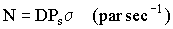(14)
where Ps= 3.092´ 1016 meters/parsec; s = effective cross section for the atom interacting with the electromagnetic radiation, and D = gas density in space (atom m-3).
Since the energy loss per collision is very small compared with unity, let us consider the so-called thin-target condition. From Eq. (12):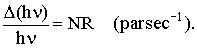(15)
Using Eq. (13) for an object at one parsec:(16)
where Ho = 0.05m/s/parsec (Hubble constant).
Since Eqs. (15) and (16) give the relative energy loss per parsec, they lead to: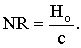(17)
Consequently, Eqs. (17) and (14) yield: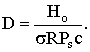(18)
The relevant cross section s is calculated for atomic hydrogen in Appendix B, Eq. (B8).  Assuming that hydrogen is uniformly distributed in space, the average density DH (atom/m3) required to produce the same redshift as the one given by the Hubble constant is obtained from Eq. (18). Combining Eqs. (B8) and (18) yields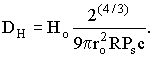19
Numerically, let us consider light coming from remote stars at a temperature of T=50 000 K. That light reaching us will travel through intergalactic space but also through some intervening galaxies. Equation 19 shows that if the average density of hydrogen is DH = 2.5 x 104 atom m-3, the redshift produced by the interstellar hydrogen considered here is then equal to the one calculated using the Hubble constant. This value corresponds to the average density of hydrogen that is required in space to satisfy the hypothesis. This density cannot be compared with the density of matter calculated using the Hubble constant and relativity because, if this density of hydrogen exists, the expansion does not exist and the determination of the density using Einstein's relativity becomes irrelevant.
This value includes the contribution of the very large gaseous nebulae or galaxies located in the line of sight or concentrated around the light source itself. Consequently, depending on the temperature of the source and the nature of the intergalactic gas, an average density of the order of 0.01 atom per cubic centimeter is sufficient to produce, on the Planck spectrum, an effect equivalent to that of a Doppler shift in agreement with the Hubble constant.

We know experimentally, that light travels in straight line in a medium as in water or air. Even after traveling one meter in air, we can calculate that photons have interacted with numerous molecules since they are delayed, as given by the index of refraction. However, after millions of collisions in air, most of the photons still maintain a parallel direction of propagation. It is easy to evaluate the angular deviation of the incident radiation due to the axial acceleration of the electron. It is known that bremsstrahlung radiation is emitted in the direction perpendicular to the acceleration of the electron. Since we are dealing with the problem of the momentum transfer of a wave train to an electron accelerated in the same direction as the incident wave, the total transverse momentum component given to the electron is zero (Newton's law). Then the sum of the transverse momentum component of the two electromagnetic wave trains re-emitted must be zero. The initial photon cannot get a large deviation when it is interacting with the hydrogen because the secondary photon generated during the interaction has too small energy and momentum to provide a sufficient recoil to the initial photon. Since the very soft bremsstrahlung emitted at 90o has momentum, the initial transmitted wave receives an extremely slight recoil in order to satisfy the law of conservation of transverse momentum components. Consequently, the transmitted radiation will be very slightly deviated from the incident direction. From geometrical considerations and equation 12, one can see that:20
In order to illustrate the smallness of q1, let us calculate it from 12 and 20 for T=20 000 K.  One finds: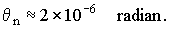21
For photons making a very large number of random collisions (i.e. n » 1012 collisions), a larger (but still extremely small) redshift is expected. The broadening of the image must have an RMS statistical width which, depending on the direction, is given by: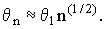22
Consequently,23
Such a random spread would make a point appear slightly fuzzy with a large telescope, of the order of magnitude observed in some quasi-stellar objects.

Let us also briefly discuss how multiple transmission interactions can make absorption lines wider and fuzzier. It is known that the redshift is proportional to the number of collisions n, but not all photons have undergone statistically the same number of collisions. Thus, the statistical spread in the number of collisions widens the absorption lines of very redshifted objects emitting blackbody radiation. Such observations are reported by Hewitt and Burbridge (6) who reports quasi-stellar objects being discovered with very broad absorption structures.

3.4 Redshift on the Sun
Let us consider the surface of our Sun. When observing its photosphere at the center of the disk, light reaching us on Earth crosses a much smaller amount of gas above the Sun’s surface than when light is coming from the limb and travels tangentially above the surface. Therefore, according to the theory described above, a larger redshift should appear near the limb.
Such a redshift near the limb has been known for about 80 years (7-12) and has been confirmed by at least 50 independent papers. It has never received a clear explanation. We have calculated that this redshift agrees quantitatively with the theoretical predictions explained above, taking into account the Sun’s temperature and the amount of gas above its surface. More details will be published on that work elsewhere.

3.5 Binary Stars
Since it is difficult to distinguish a Doppler redshift from the new redshift described above, one must look for special circumstances where the Doppler component of the phenomenon can be clearly identified. An ideal case is seen in binary stars. Celestial mechanics shows that spectral lines from components of binary stars must oscillate around the central position since the average radial velocity of the stars must be the same. Observations (9),(13) show that it is not so. Hot stars (O-stars) show a larger redshift than the cooler (A and B) stars (9),(13). When one applies the theory stated above, one sees that the extra redshift observed in the high-temperature star agrees exactly with the value deduced from such a star, taking into account the temperature and the amount of gas on its surface. This is another confirmation of the above theory.

3.6   K-Term
It is known that hot stars in the Sun’s neighborhood are moving away from us in all directions, while cooler stars do not. This phenomenon has been called K-effect. (9),(14). The apparent velocity of recession is larger for hotter stars (14). We have calculated that this effect agrees with the new redshift theory described above, knowing the amount of gas on the surface of the star and the measured temperatures of their surfaces.

3.7 Direct Detection of Bremsstrahlung Radiation
When visible light travels through gases, the mechanism described above leads to an energy loss that appears as bremsstrahlung with wavelengths several hundred meters or even kilometers long. Grote Reber, (15) with his hectometer telescope, has observed radiation from the sky in that wavelength range. He has been able to measure the map of southern sky (15) at 144 meters wavelength and is now getting data for a map of the northern sky. This radiation is compatible with the one expected from the mechanism described above.

3.8 Different Redshift in Absorption and in Emission
It has been seen [equation 12] that radiation emitted according to Planck’s law is redshifted when it is transmitted in the forward direction through an interacting gas. Emission lines, which necessarily have a much longer time of coherence than that of blackbody radiation, are also observed in the spectra of some galaxies or quasars. Their time of coherence Dt is generally much longer than that of blackbody radiation. Consequently, the emission lines due to the phenomenon described above will show a different redshift that that of the blackbody radiation.
This agrees very well with the fact that the observed absorption redshift are different from those observed in emission for all 109 quasi-stellar objects for which absorption and emission lines (of the same object) have been measured (6). It is observed that the redshift in absorption is always larger than the one in emission.

3.9 Pairs of Quasars and Multiple Absorption Redshift
Walsh, Carswell, and Weymann (16) have recently reported the discovery of a close pair of quasars having the same absorption redshift. They argue that this is extremely improbable. However, according to the present model, a double source located inside of behind the same very thick and dense nebula must show a similar redshift.
Oke (17) has reported recently that "surprisingly" the number of quasars increases as the redshift increases. Assuming the redshift mechanism described above, it is clear that an object surrounded by an extremely large amount of gas will display an important redshift and will automatically be interpreted as being at a large distance. This might explain the apparent lack of quasars at short distances.
It is also stated by Oke (17) that in some cases, different redshifted absorption lines are observed superimposed on the spectrum of one and the same star. Quasi-stellar object 0424-131 shows (6) as many as 18 different redshifts in the same spectra. We cannot ignore that 18 stars at different temperatures and surrounded by the same amount of gas would produce such a similar effect. The same phenomenon can also explain the well-observed forest of spectral Ha lines.

3.10 Implications on the Big Bang Model
In section 3.1, it is seen that an average concentration of about 10-2 particle cm-3 of gas is enough to produce a redshift that would be indistinguishable from the effect resulting from the Doppler shift attributed to the expansion of the universe. Such an average concentration of intergalactic gas is larger than usually accepted, although an almost similar concentration (103 cm-3) of gas has recently been reported (18) in some intergalactic clouds. However, the density accepted comes out of the hypothesis of a Doppler interpretation using Einstein's relativity. Such a calculation of the density of matter is space is irrelevant here, since it is based on the Doppler interpretation of the redshift, while the results obtained here are based on the energy lost due to interstellar gases which is a Non-Doppler interpretation. Therefore, the density calculated is erroneous if the universe is not expanding, as it seems to be.
The actual density of gases observed lead to higher densities that predicted. Mean concentrations of the order of one particle per cm-3 have been measured in galaxies. Consequently, radiation having a path across the diameter of a galaxy and traveling through such a large density of gas would undergo a measurable spectral redshift. Furthermore, Scoville and Sanders (19) have measured huge molecular clouds with masses up to 106 solar masses and diameters up to 80 parsecs, giving a density of 200 hydrogen molecules cm-3. The amount of gas discovered inside and outside galaxies is becoming increasingly important. Should we expect new discoveries? Is this related to the missing mass that would stabilize galaxies? From the increasing rate of discoveries of gas in space, it does not appear improbable that a particular light path will have its light interacting a sufficiently large number of times in the forward direction to produce an important atomic or molecular redshift.
However, when the redshift obtained from the measurement of absorption lines is different from the one deduced from emission lines, as appears to be the results reported by Hewitt and Burbidge (6) and Arp and Sulentic (20), it must be concluded that this is another agreement with this paper. Many other interesting observations (21) should be considered in order to find new proofs of the new model that lead to a new interpretation of the redshift. This is consistent with the observation that large-scale structures of the universe get larger (22) so that "… theorists know of no way such a monster could have condensed in the time available since the Big Bang … "(22). This new Non-Doppler redshift opens a new field of investigation related to bremsstrahlung.

4. Laboratory Verification of Such a Redshift.
Some laboratory experiments could be considered in order to prove actual redshifts in gases. Could the necessary conditions to generate redshifts be produced in a lab in order to demonstrate this phenomenon? Could this phenomenon be measured when radiation passes through air at atmospheric pressure? It is well known that laser light can be tested very efficiently through great distances in air in order to detect micro-redshifts. We have seen that a more important redshift is produced when the length of coherence is short. Therefore, the long time of coherence, which is a fundamental characteristic of lasers, is specially inappropriate for the measurement of redshifts in gases. Therefore, such a test is useless, because the time of coherence of laser radiation is much too long.
Although narrow absorption lines are more difficult to measure accurately, they might help to solve this problem. However, further considerations show that they do not seem to offer much hope of yielding a positive measurement when transmitted through long distances in air. Since the average distance between molecules at atmospheric pressure is much smaller than that of the length of coherence of the radiation used, the electromagnetic field is applied in phase on many molecules. Therefore, the photon momentum is distributed simultaneously on the total mass of the molecules. A similar phenomenon is explained by Feynmann, Leighton, and Sands, (23) for Thompson scattering of light in air. This phenomenon also corresponds to a description of the Mössbauer effect at low temperature, where the atoms recoil in phase. Consequently, the bremsstrahlung produced in a gas at high pressure is extremely small, because the radiation is simultaneously accelerating many electrons in phase within the length of coherence of the radiation. Therefore, the combined mass of all the electrons emits much less bremsstrahlung radiation. Such an experiment would have to be done at pressures lower than atmospheric, but the path length would have to be correspondingly long in order to produce a detectable signal.

<><><><><><><><><><><><>
Appendix A:
Time of Coherence or Pulse Duration of Blackbody Radiation.

There are several methods of calculating the time of coherence of electromagnetic radiation. Whether the electromagnetic radiation comes from an excited state having a lifetime of 10-8 s. or has a wide Planck distribution, the energy emitted has a time of coherence that can be calculated from a Fourier analysis of its emission spectrum (24). The pulse shape of the wave emitted by a black body is given by the inverse Fourier transform f(t) of Planck’s function. The Planck spectrum giving the amplitude density dA(n) emitted per unit area as a function of frequency n is: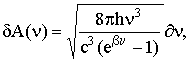A1
Where b = h/(kT); k=Boltzmann’s constant; T=Kelvin temperature.
A pulse having Planck’s spectrum F(n )=d A(n ) will have an amplitude f(t) as a function of time t given byA2
One considers only the real part of A2 one obtains: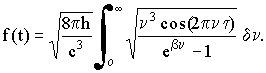A3
Only the real part contains energy. The imaginary part contains the phase relationship.
It can be shown that the integral of A3 is approximately equal to: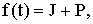A4
Where J=-24p h/(c3t4) and
P=[8p5h/(c3b4)][csch2(2p2t/b )][2+3csch2(2p2t/p )];
The limit f(t)for t=0 is:A5
The inverse Fourier transform A4 can be written in a normalized form having an amplitude of one at the origin, by taking the ratio R such that: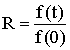A6

In order to determine the properties of this function, let us use the variables :and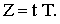A7
The normalized Fourier transform R becomes: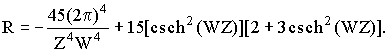A8
Equation A8)is an analytical function having an excellent approximation. It gives a description of the phenomenon (25). A very accurate result (which is quite similar) can be obtained using the numerical Fourier transform. The Planck function is plotted as curve A in figure 1 and its numerical inverse Fourier transform is plotted in amplitude as curve B and in energy as curve C. From this numerical inverse Fourier transform, one finds that the pulse width (Dt) at half height (in energy) having the Planck spectra is: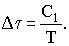A9
where C1=2.183 x 10-12
The most probable frequency n max emitted in Planck’s spectrum is:A10
where C2=5.88 ´ 1010 s-1K-1
The time Dt described in A9 is characteristic of the duration of the wave packet emitted by the blackbody at a given temperature T and therefore, it is mainly influenced mainly by the most probable frequency component nmax emitted. Consequently, the effective pulse duration of the most important frequency component nmax is considered to be the effective pulse duration calculated above for nmax. That pulse duration is inversely proportional to the temperature of the emitting surface as seen in A10.
We also wish to show that, within a spectrum at a given temperature, Planck’s quantum postulate leads to different values of pulse duration depending on the wavelength considered. According to Planck, the blackbody spectrum is composed of a finite number of frequencies (26) resulting from standing waves emitted from a cavity. These frequencies are given by the expression: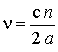A11
where a= length of the cavity, and n = 1, 2, 3, 4, . . .
As a consequence of the finite number of frequencies, Planck’s spectrum must be represented as a sum of discrete frequencies. All these frequency components must be coherent, and the signal must be non-interrupted during the full interval of time of emission, since it is necessary to reproduce exactly the Planck’s spectrum.
Let us consider two independent emitters of electromagnetic radiation at different frequencies n1 and n2. These emitters produce a continuous wave with the same amplitude (therefore the same energy) during the same period of time. Each electromagnetic wave crosses atoms of gases, each having a resonant absorption line located at the same frequency as that of the wave. The absorption mechanism is such that the electromagnetic radiation is absorbed when an atom interacts. This is the consequence of the quantization of atomic states. The time of interaction of the wave Dt is the one required to accumulate enough energy to excite the atom. After all the energy of the beam is absorbed by the gas, one finds that the product of the time Dt during which the wave is interrupted (which is the time of interaction) times the number of atoms excited per second is equal to the unit time.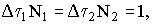A12
Where Dt1= time of interruption of the wave due to absorption by atoms N1; Dt2= time on interruption of the wave due to absorption by atoms N2; N1= number of atoms of gas N1; N2 = number of atoms of gas N2.
From the law of energy conservation: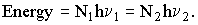A13
Combining A12 with A13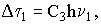A14
Where C3 = (Dt2)/(hn2) is a constant for a given experimental condition.
Equation A14 shows that within a given spectrum of blackbody radiation, the effective wave duration Dt is proportional to its frequency n .
There remains to combine A9 and A14. Let us examine A14. For n =nmax we find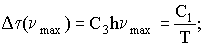A15
Therefore,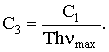A16
Equation A10 in A16 gives,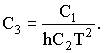A17
Substituting in A14 yields,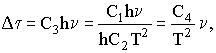A18
Where C4= C1/C2= 3.71 ´ 10-23 s2K2.
One must finally recall that these conclusions depend directly on the fact that the spectrum is made of discrete values following Planck’s quantum postulate described in A11. Consequently, A18 gives the relation between the time of coherence Dt at any frequency n in the case of blackbody radiation.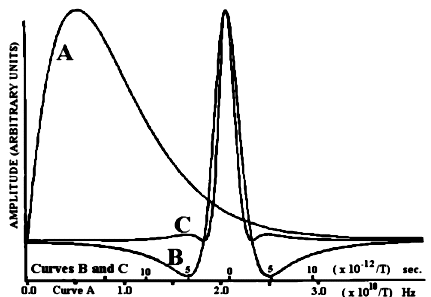Figure 1
Curve A is the amplitude of the blackbody radiation (Planck function) as a function of frequency n at temperature T. Curve B shows the amplitude of the inverse Fourier transform of A as a function of time t. The scale can be ajusted at any temperature T. Curve C is the square of B. It gives the energy at any temperature T as a function of time t.

<><><><><><><><><><><><>
Appendix B:
Relevant Cross Section of Hydrogen.

Let us calculate the cross section s, for which the incident wave gives its energy to the atom through polarization and deduced from the mechanism of transmission of radiation through gases. This cross section is obtained using the same considerations as for calculating the dielectric constant of gases.
Let us consider hydrogen at an extremely low pressure, a condition prevailing in outer space. If the radiation undergoes only one collision per week, it is then completely inappropriate to consider that the velocity of propagation is simply slightly smaller than c. In that case, it is evident that the index of refraction is exactly one during six of the seven days and also 23 of the 24 hours of the exceptional day, and so on during the last minute, second, and its fraction, until one has to arrive at a non zero interval of time of interaction between the absorption of radiation and its reemission.
Between absorption and reemission, the atoms must then momentarily retain the absorbed energy and momentum. This mechanism leads to identical times of propagation whether one considers a change of index of refraction or individual collisions with delayed energy reemission. Therefore, there is certainly a delay during the interaction of the photon with the particle. The latter model, however, is closer to the atomic nature of matter.
It is known that the index of refraction n of a transparent medium is the ratio: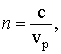B1
where vp = phase velocity of the radiation in the medium.
It is also known that the velocity of propagation of energy corresponds to the group velocity vg. In order to be able to point out the parameters involved in the evaluation of the cross section s, let us calculate the group velocity of light in atomic hydrogen using a method analogous to the one suggested by Feynmann, Leighton, and Sands (23) to study the dielectric constants of gases that similarly depend on their polarizability.
It is well known (23) that the index of refraction for visible light in hydrogen is: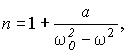B2
where a = Ne2/(2eomo); wo= angular velocity of a resonant electron in hydrogen; w = angular velocity of the incoming radiation; N = number of atoms per m3.
Since we know that the energy is transmitted with a velocity equal to the group velocity vg, it can be demonstrated using Feynmann method (23) thatB3
Full details have been given elsewhere 23, 25. From quantum mechanical considerations, there is a relatively minor correction for the polarizability of hydrogen. It is known that the coefficient 8 in equation B3 must be replaced by 9, giving: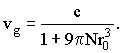B4
Since B4 is valid only in the energy range wo2 >>w 2, let us verify that this approximation is valid in the energy range given by the usual blackbody radiation spectrum. Let us compare the index of refraction n of hydrogen predicted by B4 with experimental data. At atmospheric pressure, we have found that B4 gives n = 1.00012. The best experimental value available is that of the H2 molecule, which is predicted to be about the same. In H2 the measured value is n = 1.00013 for visible light. This is in excellent agreement with equation B4 which is thus valid for visible and longer wavelengths.
Equation B4 has been derived here in order to point out that the quantity 9pro3 added to the denominator has the dimension of a volume. The total volume is directly proportional to the density of atoms N located on the radiation path. Consequently, radiation behaves as if each atom adds an extra virtual volume 9pro3 in the light path.
Therefore equation B4 shows that the time taken for the electromagnetic radiation to cross a given distance inside a volume containing N atoms per cubic meter is equal to the time the light would take in a vacuum to cross the same volume, to which one must add a virtual volume VV per particle, which is equal to: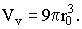B5
This last result may be conveniently used to support the hypothesis of absorption and reemission of radiation (as suggested previously) since the total virtual volume is proportional to the number of atoms times the virtual volume per atom VV.Since the probability of finding an electron around a hydrogen atom in the ground state being spherically symmetrical, let us calculate the cross section sH for hydrogen having a virtual radius rv and virtual volume VV. We have: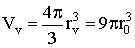B6
and sinceB7
equations B6 and B7 give the cross section sH for hydrogen. Consequently,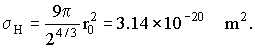B8
Equation B4, which leads to B8, shows that within the approximation used to obtain B4, sH is wavelength independent. This result is not unique and has been obtained previously (23) in comparable cases for the dielectric constant of gases. The predicted result, leading to a constant virtual volume different from zero when 0, is verified experimentally, since the dielectric constants of gases are larger than of the vacuum (k=1) even if w becomes vanishingly small.
It is known that the virtual volume is a function of the polarizability of the atom. One must not be surprised that the cross section s found here remains finite and 0 when 0. This cross section is of course completely different from the scattering cross section used in Thomson scattering. It is related to the index of refraction for which light propagates in straight line.
Finally, for the case of a single free electron, the Thomson scattering cross section may be considered. It is s =6.65 x 10-29 m2, which is about nine orders of magnitude smaller than that obtained above for the hydrogen atom and consequently has no practical interest in this paper.

New Verification and Supporting Evidence.
Several new papers with experimental proofs supporting the energy loss of photons due to the traces of hydrogen in space have been published more recently. For example, a paper entitled: The Cosmological Constant and the Red Shift of Quasars (27), explains the consequences of a redshift due the traces of hydrogen in outer space. Furthermore, another paper entitled: Non-Doppler Redshift of Some Galactic Object" (28) shows that the difference of redshift between the components of binary stars systems can only be explained by the difference of temperature responsible for the change of coherence of blackbody radiation as explained above. Furthermore, that same paper shows that the K effect and other astronomical observations require that photons are redshifted when moving through traces of hydrogen gas. Also, the solar atmosphere shows a redshift which varies as a function of the radial distance as seen from he Earth.  That is explained in the paper(29): "Redshift of Spectral Lines in the Sun's Chromosphere". That redshift remained unexplainable until it was realized that the hydrogen in the solar atmosphere has exactly the correct concentration to explain its redshift (as explained above). Finally, various other descriptions of that phenomena have been presented (30).

<><><><><><><><><><><><>
==================== =====================
Back cover of the Book (Printed in June 1981)
A New Non-Doppler Redshift.

There are now 109 QSO’s for which the redshift value Z has been determined independently both in emission as well as in absorption.  In all 109 cases, the emission redshift is different from the absorption shift  (for one and the same object).
This is clearly contrary to the Doppler hypothesis.
Many more observations lead to results, which are incompatible with the interpretation that redshifts are due to relative velocity.
This book shows that taking into account the change in momentum of the electrons of gas molecules scattering light in space leads to bremsstrahlung and a slightly inelastic forward scattering.
This is the first Non-Doppler redshift theory, which when combined with the usual Doppler phenomenon, would explain consistently all spectral shifts observed in astronomy.
------------------------------------ ------------------------------------
References.

1   I. F. Boekelheide, Ph. D. Thesis, State University of Iowa, June 1952.
2   P. E. Cavanaugh, Phys. Rev. 87, 1131, 1952.
3   J. M. Jauch and F. Rohrlich, The Theory of Photons and Electrons, Addison-Wesley, Cambridge, Mass, 1955.
4   L. I. Schiff, Quantum Mechanics, 2nd ed. p.417. McGraw-Hill, 1955.
5   G. Field, H. Arp, and J. N. Bahcall, The Redshift Controversy, W. A. Benjamin, Inc., Reading, Mass, 1973.
6   A. Hewitt and G. Burbidge, Astrophys. J., Suppl. Ser. 43, 57, 1980.
7   J. Halm, Astron. Nachr. 173, 273, 1907.
8   W. S. Adams, Ap. J. 31, 30, 1910.
9   E. Finley-Freundlich, Phil. Mag. 7, 303, 1954.
10  L. A. Higgs, Mon. Not. R. Astron. Soc. 121, 167, 1960.
11  F. Cavallini, G. Ceppatelli, and A. Righini, Astron. Astrophys. 143, 116, 1985.
12  E. Schatzman and C. Magnan, Astron. Astrophys. 38, 373, 1975.
13  L. V. Kuhi, J. C. Peker, and J. P. Vigier, Astron. Astrophys. 32, 111, 1974.
14  C. W. Allen, Astrophysical Quantities, 3rd ed. The Atlone Press, p.253, 1973.
15  G. Reber, Endless, Boundless, Stable Universe, University of Tasmania, Australia, Occasional paper No: 9, 1977.
16   D. Walsh, R. F. Carswell, and R. J. Weymann, Sky & Telescope 58, 427, 1979.
17   J. B. Oke, Sky & Telescope, 58, 508, 1979.
18  W. L. Sargent, P. J. Young, A. Boksenberg, and D. Tytler, Abstract of Astrophysics. J., Suppl. Ser. 42, 41, 1980.
19  N. Z. Scoville and D. B. Sanders, Physics Today 33, 17, 1980.
20  H. Arp and J. W. Sulentic, Astrophys. J. 229, 496, 1979.
21  H. Arp, Quasars, Redshifts and Controversies, Interstellar Media, Berkeley, Calif., 1987.
22  M. M. Waldrop, Science 238, 894, 1987.
23  R. P. Feynmann, R. B. Leighton, and M. Sands, Lectures on Physics, Addison-Wesley, Reading, Mass., 1964.
24  M. Françon and S. Slansky, Cohérence en optique, Centre national de la recherche scientifique, Paris, 1965.
25  P. Marmet, "A New Non-Doppler Redshift", (book), Département de Physique, Université Laval, Québec, Canada, 1981.
26  R. Eisberg and R. Resnik, Quantum Physics of Atoms, Molecules, Solids, Nuclei and Particles,  Wiley, 1974.
27  P. Marmet, The Cosmological Constant and the Redshift of Quasars" IEEE Transactions on Plasma Science, Vol. 20 No. 6, p. 958-964, 1992.
28  P. Marmet, Non-Doppler Redshift of Some Galactic Objects. IEEE Transactions on Plasma Science,Vol. 18, No. 1, p. 56-60, 1990.
29  P. Marmet, Redshift of Spectral Lines in the Sun's Chromosphere, IEEE Transactions on Plasma Science, Vol. 17. No. 2, p. 238-244, 1989.
30  P. Marmet, Big Bang Cosmology Meets an Astronomical Death. 21st Century, Science and Technology, Vol 3, No: 2, 1990.

<><><><><><><><><><><><>